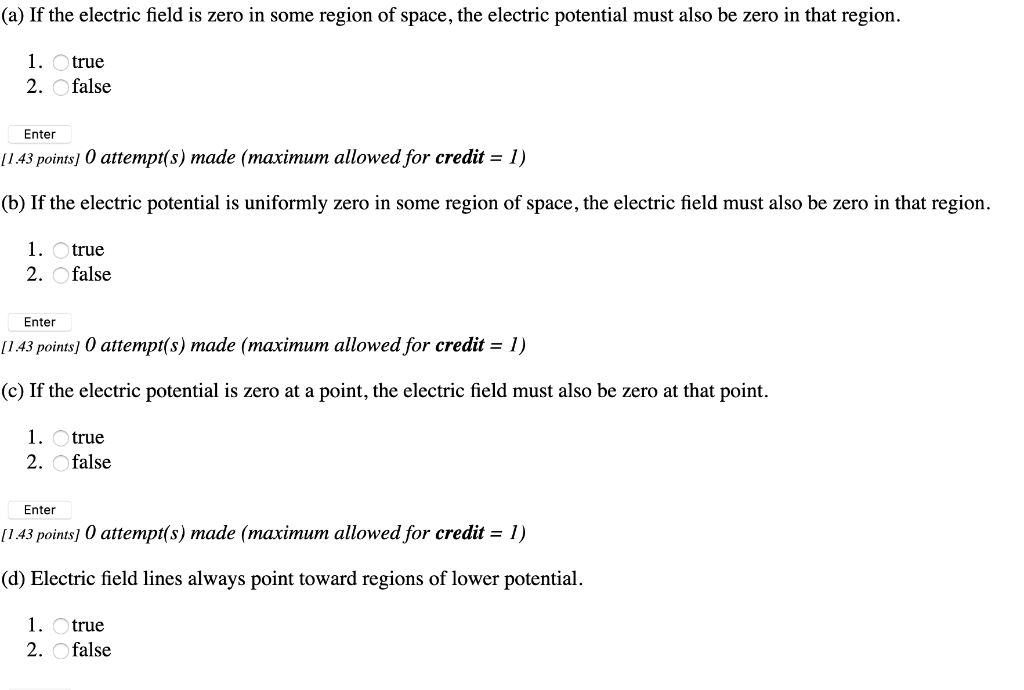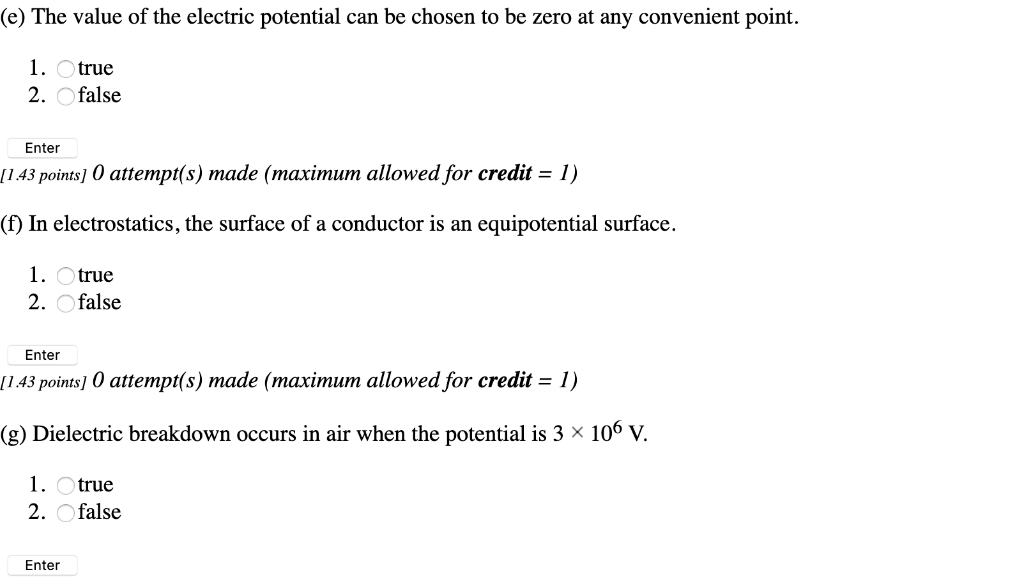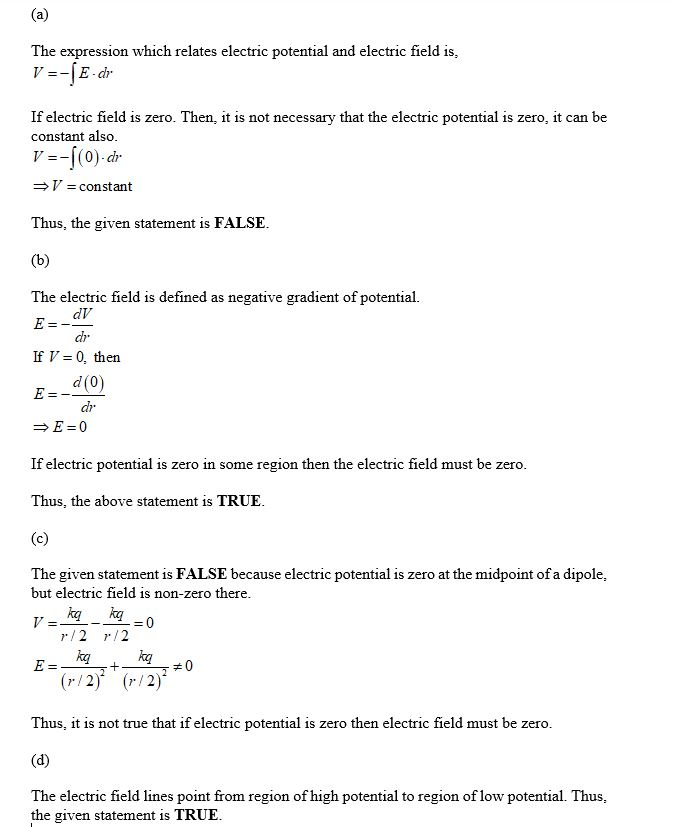# When the electric field is zero at a point. the potential must also be zero there.(a) If the electric field is zero in some region of space, the electric potential must also be zero in that region 1. true 2. false Enter 143 роints] 0 attempt(s) made (тахітит аllowed for credit — 1) (b) If the electric potential is uniformly zero in some region of space, the electric field must also be zero in that region 1. true 2. false Enter 143 рoints) 0 attempt(s) made (таximит allowed for credit – 1) (c) If the electric potential is zero at a point, the electric field must also be zero at that point. 1 true 2 false Enter [143 рoints] 0 attempt(s) made (таximит allowed for credit — 1) (d) Electric field lines always point toward regions of lower potential 1. true 2. false (e) The value of the electric potential can be chosen to be zero at any convenient point. 1 true 2. Ofalse Enter 1 43 роints] 0 attempt(s) тade (тахітит allowed for credit — 1) equipotential surface (f) In electrostatics, the surface of a conductor is an 1. Otrue 2. Ofalse Enter П43 рoints] 0 attempt(s) тade (тахітит allowed for credit — 1) (g) Dielectric breakdown occurs in air when the potential is 3 x 106 V. 1. Otrue 2. Ofalse Enter

The expression which relates electric potential and electric field is, V =- E-dr If electric field is zero. Then, it is not necessary that the electric potential is zero, it can be constant also. V=-10) dr V = constant Thus, the given statement is FALSE. (b) The electric field is defined as negative gradient of potential. If V = 0, then d(0) E =- dr = E=0 If electric potential is zero in some region then the electric field must be zero. Thus, the above statement is TRUE. (c) The given statement is FALSE because electric potential is zero at the midpoint of a dipole. but electric field is non-zero there. 1/2 1/2 = 0 Thus, it is not true that if electric potential is zero then electric field must be zero. (d) The electric field lines point from region of high potential to region of low potential. Thus, the given statement is TRUE.